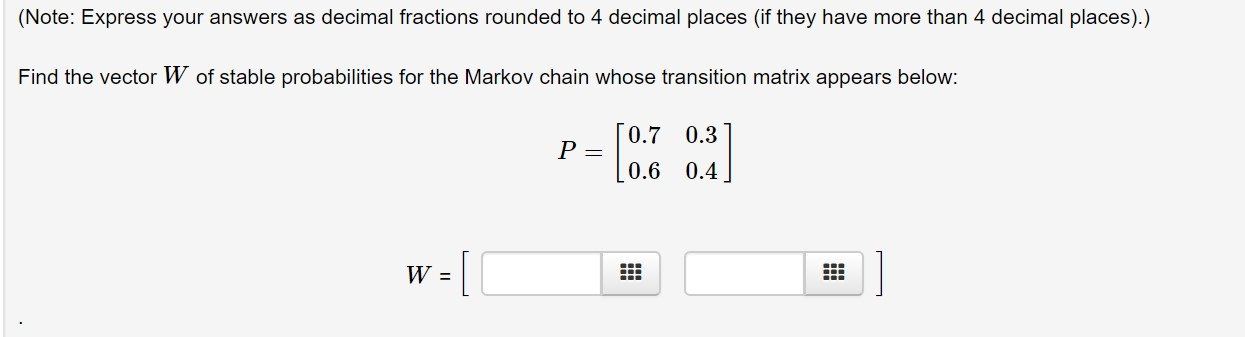# (Note: Express your answers as decimal fractions rounded to 4 decimal places (if they have more than 4 decimal places).)Find the vector W of stable probabilities for the Markov chain whose transition matrix appears below:0.70.30.6 0.4

Question
1 viewshelp_outlineImage Transcriptionclose(Note: Express your answers as decimal fractions rounded to 4 decimal places (if they have more than 4 decimal places).) Find the vector W of stable probabilities for the Markov chain whose transition matrix appears below: 0.7 0.3 0.6 0.4 fullscreen
check_circle

Step 1

Given transition matrix is,

Step 2

Then vector W of stable probabilities fo...

### Want to see the full answer?

See Solution

#### Want to see this answer and more?

Solutions are written by subject experts who are available 24/7. Questions are typically answered within 1 hour.*

See Solution
*Response times may vary by subject and question.
Tagged in

### Math